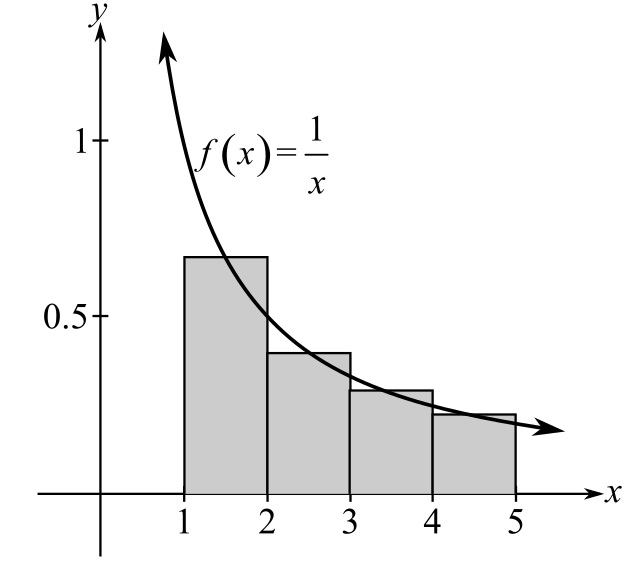Chapter 5.6, Problem 2E### Calculus: An Applied Approach (Min...

10th Edition
Ron Larson
ISBN: 9781305860919

#### Solutions

Chapter
Section### Calculus: An Applied Approach (Min...

10th Edition
Ron Larson
ISBN: 9781305860919
Textbook Problem
1 views

# Approximating the Area of a Plane Region In Exercises 1-6, use the rectangles to approximate the area of the region. Compare your result with the exact area obtained using a definite integral. See Example 1. f ( x ) = f 1 x , [ 1 , 5 ]To determine

To calculate: The area bounded by the function f(x)=1x between interval [1,5] by mid-point rule. Also compare this approximate area with exact area using definite integral.Explanation

Given Information:

The provided function is f(x)=1x. Area of this function is calculated between the interval [1,5] as shown below:

Formula used:

The approximate area of any definite integral baf(x)dx by the use of midpoint rule is calculated by the use of following steps.

Step1: First divide the provided interval of the function into n sub intervals by the use of formula;

Δx=ban where [a,b] are the intervals and n is the subinterval value and Δx is the width.

Step2: Use above subinterval find the midpoint of each sub interval.

Step3: Final step is to obtain approximate area by calculating function f at each mid-point from the use of formula;

abf(x)dxban[f(x1)+f(x2)+f(x3)+....+f(xn)].

Calculation:

Consider the provided function f(x)=1x. The graph of provided function is shown below:

In provided graph, interval [1,5] is divided into 4 rectangle.

Now, put n=4, a=1 and b=5 to find the width of each rectangle as;

Δx=514=1

Width Δx of each rectangle will be 1.

So, the interval [1,5] will be divided into 4 subintervals as;

[1,2],[2,3],[3,4],[4,5].

Now, the value of midpoint of each rectangle is calculated as;

[x1,x2]=x1+x22 where x1,x2 are the upper value and lower value of the sub-interval

So, for first rectangle [1,2] mid-point is;

1+22=1.5

Similarly, for second rectangle [2,3] mid-point is;

2+32=2.5

For third rectangle [3,4] mid-point is;

3+42=3.5

For last rectangle [4,5] mid-point is;

4+52=4

### Still sussing out bartleby?

Check out a sample textbook solution.

See a sample solution

#### The Solution to Your Study Problems

Bartleby provides explanations to thousands of textbook problems written by our experts, many with advanced degrees!

Get Started

#### Sketch the graphs of the equations in Exercises 512. 2y+x=1

Finite Mathematics and Applied Calculus (MindTap Course List)

#### Evaluate the limit. limxe2xe2xln(x+1)

Single Variable Calculus: Early Transcendentals, Volume I

#### Evaluate the integral. 30. x32x2+2x5x4+4x2+3dx

Single Variable Calculus: Early Transcendentals

#### How many ft2arein140yd2?

Elementary Technical Mathematics

#### True or False:

Study Guide for Stewart's Multivariable Calculus, 8th